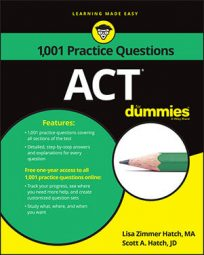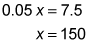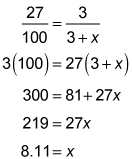##### ACTWhen you set one ratio equal to another ratio, you've created a proportion. Understanding how to solve a proportion will come in handy on the ACT Math exam.

In the following practice questions, you're asked to solve a proportion using cross-multiplication and then to find the best proportion of concentrate to water to create a perfect glass of orange juice.

## Practice questions

1. If basis points are defined so that 5 percent is equal to 100 basis points, then 7.5 percent is how many basis points greater than 5.5 percent?

A. .04 B. 40 C. 400 D. 4,000 E. 40,000

2. A local diner prepares orange juice beverage every morning by adding orange juice concentrate to water. The owner has determined that the best beverage mix results when 27 percent of the orange juice beverage is made up of orange juice concentrate. How many liters of water to the nearest full liter should be added to 3 liters of concentrate to create the optimum mixture of orange juice beverage?

A. 8 B. 11 C. 14 D. 17 E. 20

## Answers and explanations

1. The correct answer is Choice (B). Don't spend a bunch of time worrying about what basis points are in this question. They're just units of measurement.

This question really wants to see what you know about proportions. You know that 5 percent equals 100 basis points. You can use a proportion to find out how many basis points 7.5 percent equals.

Cross-multiply:You could figure out the basis points for 5.5 percent in the same way, but you don't have to take the time to do the calculations. All of the answer choices begin with 4, so you know that the basis points for 5.5 percent begins with the value that results in a number that begins with 4 when it's subtracted from 150. The value also has the same decimal placement as 150 because 7.5 and 5.5 have the same decimal placements. So you know that the number of basis points for 5.5 percent is 110, and the difference between the two basis points values of 150 and 110 is 40.

2. The correct answer is Choice (A).

The ultimate mix with 3 liters of concentrate should result in a beverage that's 27 percent orange juice concentrate and the rest water. Percentages can be expressed as ratios. The best way to solve the problem is to set up a proportion with amount of concentrate on top and the total liquid in the beverage on the bottom on both sides of the equal sign. The left side of the equation shows the portion orange juice concentrate makes of the final beverage:

You put 27 over 100 because percent means "per hundred." On the right side of the proportion, you put the amount of concentrate over the total amount of the beverage mixture. The question tells you that the amount of concentrate is 3 liters. The total amount of beverage is concentrate plus water, or 3 + x. Set up the proportion and solve for x:Round to the nearest whole liter to find that the diner should mix 8 liters of water with the 3 liters of concentrate.